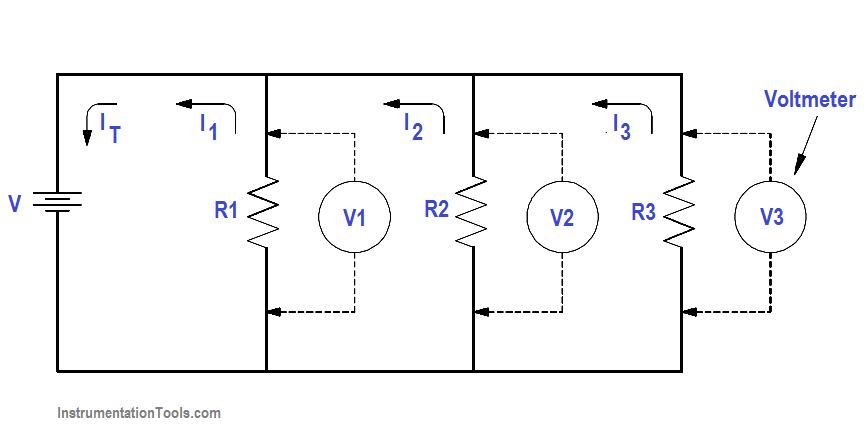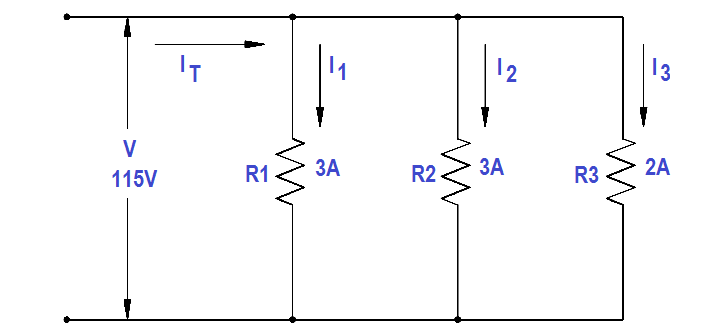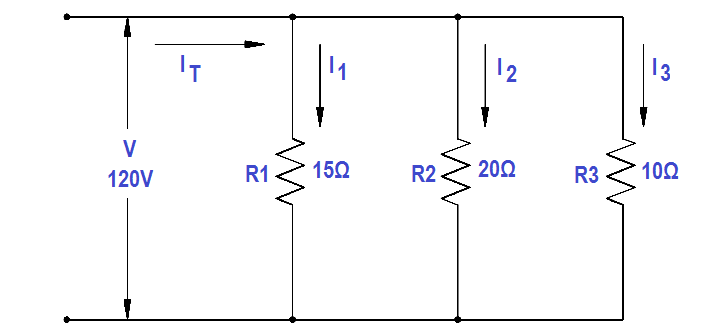# Parallel Circuit Current Calculations

The sum of the currents flowing through each branch of a parallel circuit is equal to the total current flow in the circuit.Using Ohm’s Law, the branch current for a three branch circuit equals the applied voltage divided by the resistance as shown in below equations.Example 1:

Two resistors, each drawing 3A, and a third resistor, drawing 2A, are connected in parallel across a 115 volt source (Figure 23). What is total current?Figure 23 Example 1 Parallel Circuit

IT = I1 + I2 + I3

IT = 3 + 3 + 2 = 8 A

Example 2:

Two branches, R 1 and R2 , are across a 120 V power source. The total current flow is 30 A (Figure 24). Branch R1 takes 22 amps. What is the current flow in Branch R2 ?Figure 24 Example 2 Parallel Circuit

Solution :

IT = I1 + I2

I2 = IT – I1

I2 = 30 -22 = 8A

Example 3:

A parallel circuit consists of R1 =15Ω, R2 =20Ω and R3 =10Ω, with an applied voltage of 120 V (Figure 25). What current will flow through each branch?Figure 25 Example 3 Parallel Circuit

Solution :

I1 = V/R1 = 120/15 = 8A

I2 = V/R2 = 120/20 = 6A

I3 = V/R3 = 120/10 = 12A

IT = I1 + I+ I

IT = 8 + 6+ 12 = 26 A